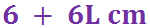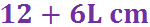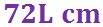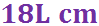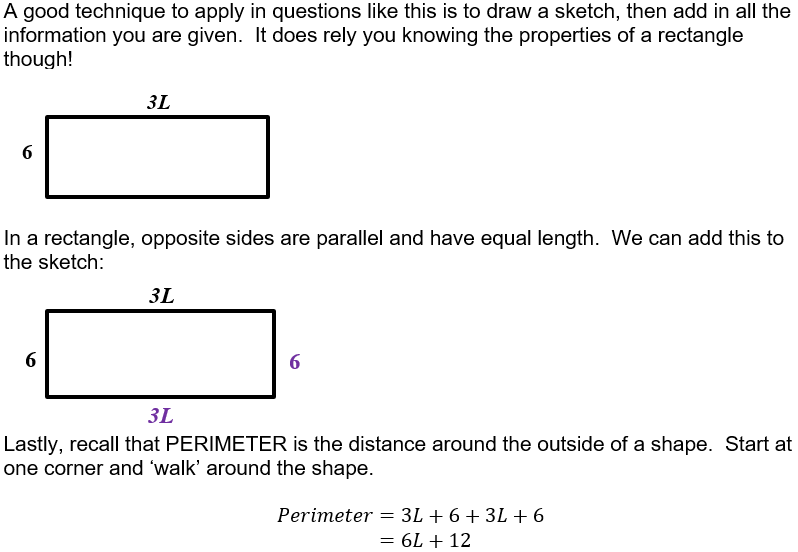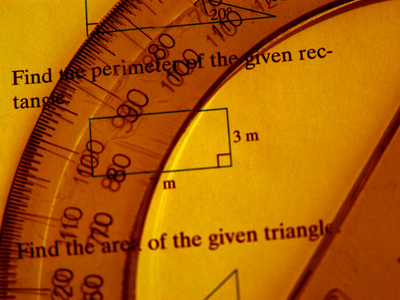Having a good grasp of numbers is important in life!

# Algebra 02 (F)

## Quiz About Indices and Simplifying Expressions

This GCSE Maths quiz will test more of your Algebra skills. You will need to know the Laws of Indices to help simplify expressions.

Sometimes you are asked to EXPAND an expression – part of the expression will be in brackets, and it is multiplied by something just outside the bracket. You need to remember to multiply EACH term that is inside the bracket.

This quiz also includes EQUATIONS. An equation is formed when an expression is given a value: that is, the expression equals something. Solving an equation involves finding out what value the letter has. When solving equations try to think of it as old-fashioned weigh scales – it needs to stay balanced, so you must do the same thing to both sides. Write down each step, using a new line for each stage of working.

Algebra can be used in problem-solving questions, often those involving shapes. Make sure you know the properties of common shapes such as rectangles, squares and triangles. It is always a good idea to sketch out the situation if a picture is not already drawn for you.

Question 1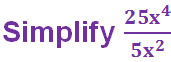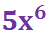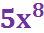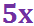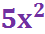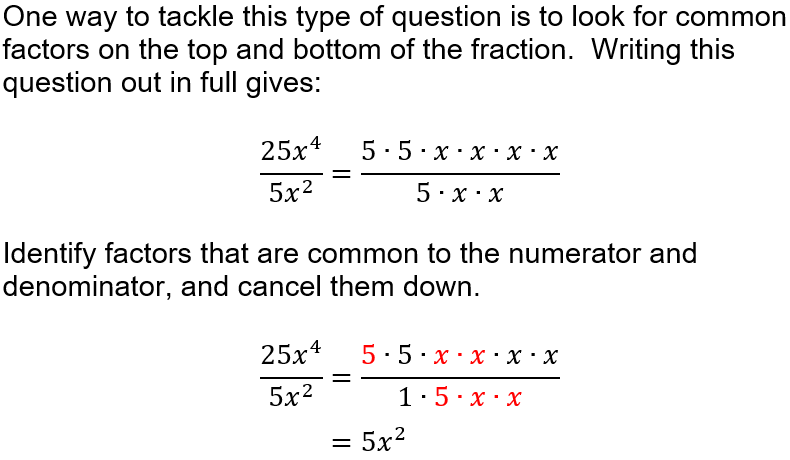Question 2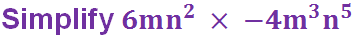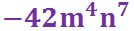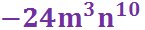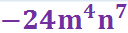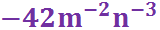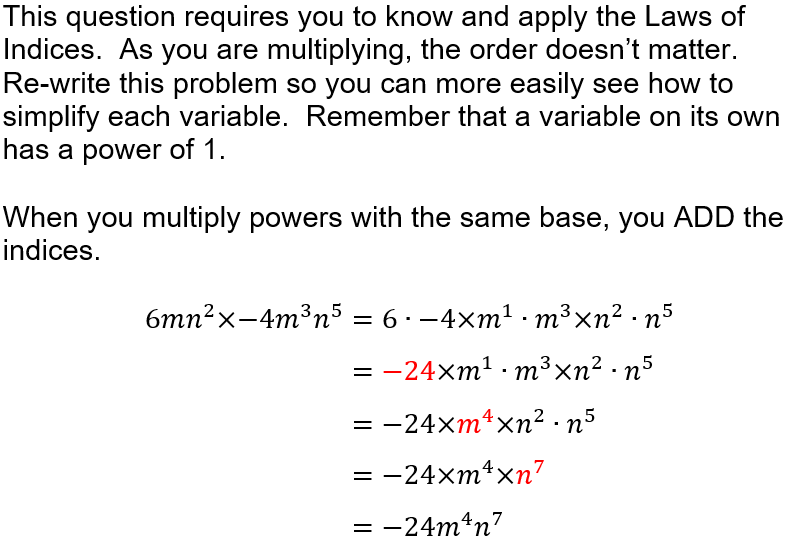Question 3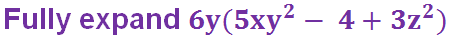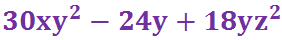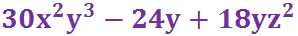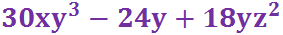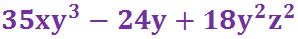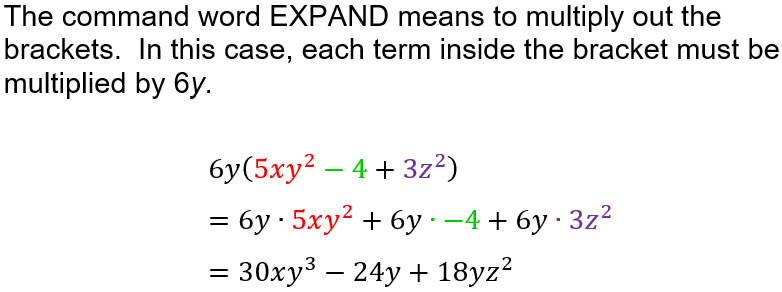Question 4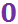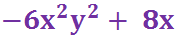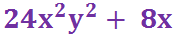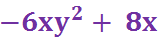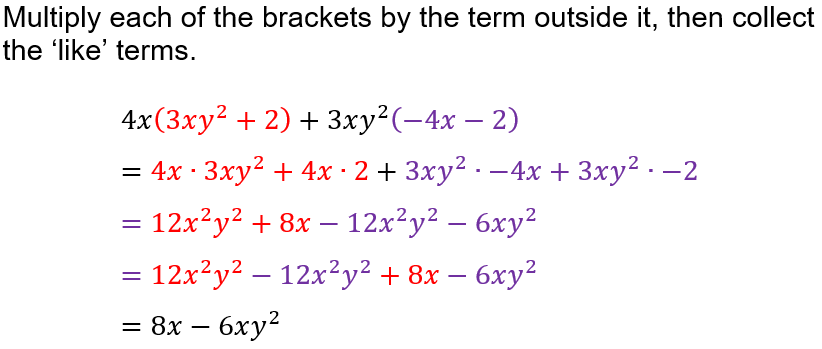Question 5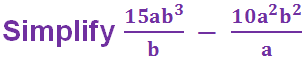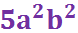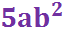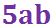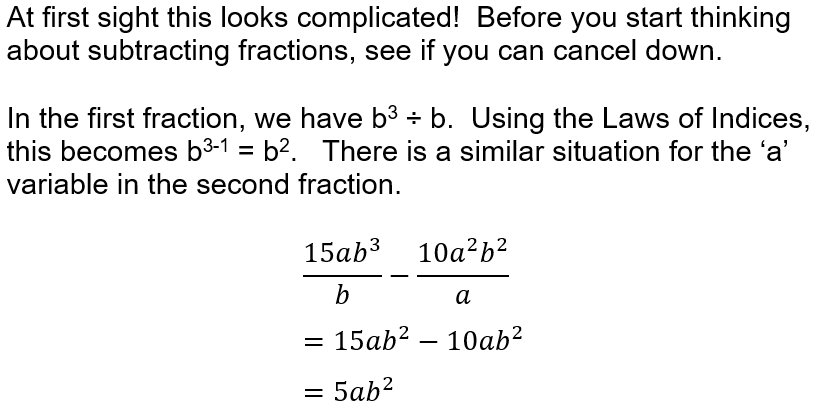Question 6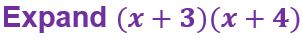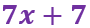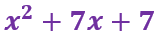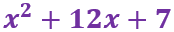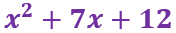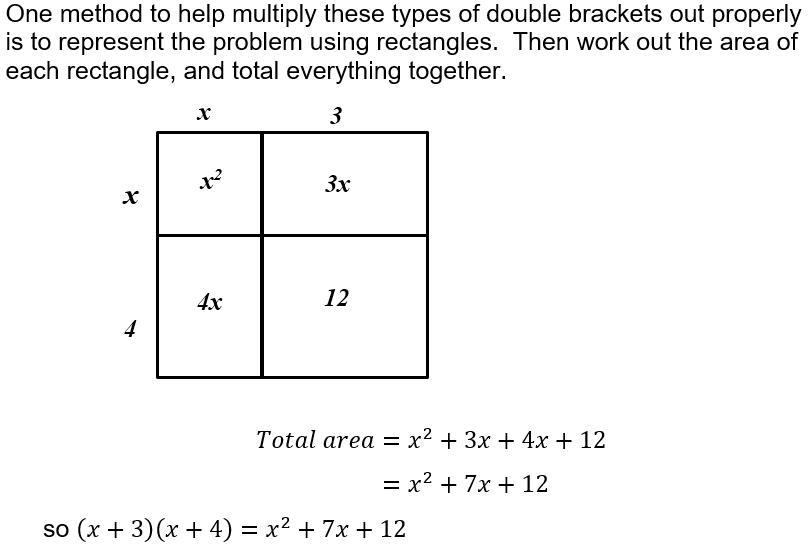Question 7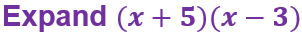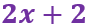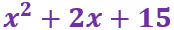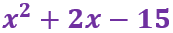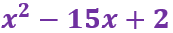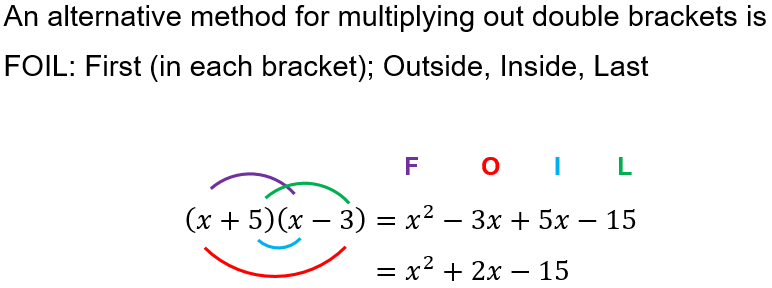Question 8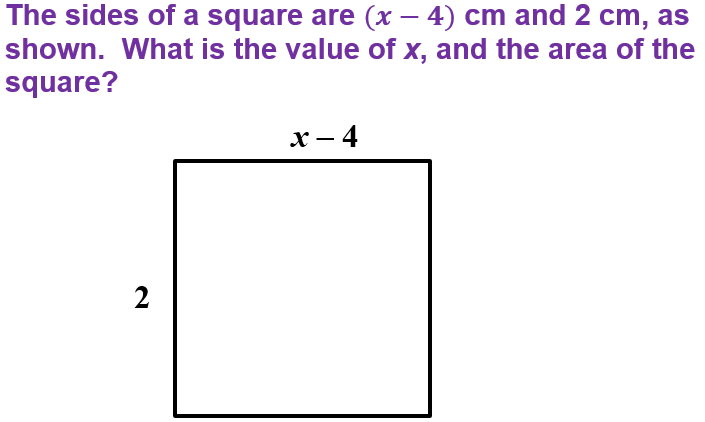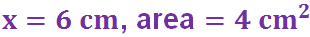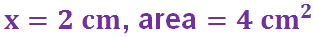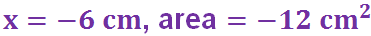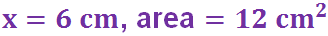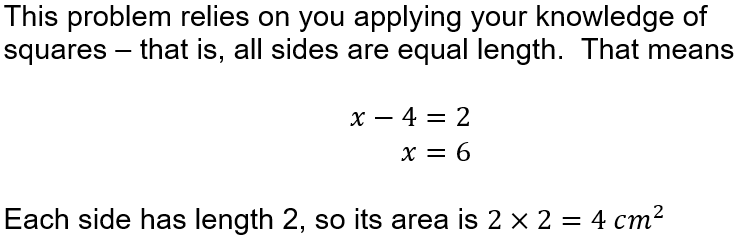Question 9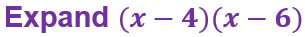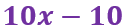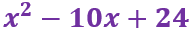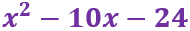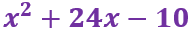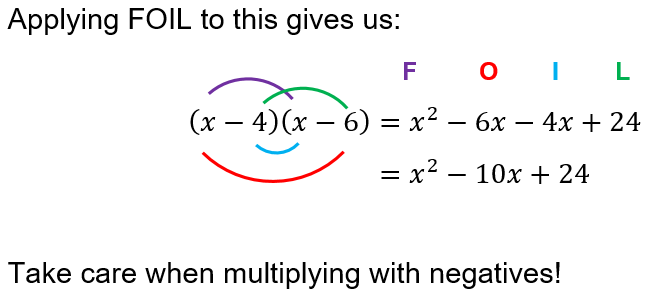Question 10